JMSLTM Numerical Library 7.2.0
com.imsl.stat

## Class DiscriminantAnalysis

• ```public class DiscriminantAnalysis
extends Object```
Performs a linear or a quadratic discriminant function analysis among several known groups.

`DiscriminantAnalysis` allows linear or a quadratic discrimination and the use of either reclassification, split sample, or the leaving-out-one methods in order to evaluate the rule. One or more observations can be added to the rule during each invocation of the `update` method.

`DiscriminantAnalysis` results in the measure of distance between the groups,(see `getMahalanobis` method), a table summarizing the classification results, (see `getClassTable`), a matrix containing the posterior probabilities of group membership for each classified observation, (see `getProbability`), the within-sample means, (see `getMeans`) and covariance matrices computed from their LU factorizations, (see `getCovariance`). The linear discriminant function coefficients are also computed, (see `getCoefficients` method).

All observations can be input during one call to the `update` method; this has the advantage of simplicity. Alternatively, one or more rows of observations can be input during separate calls to `update`. This does not require all observations be memory resident, a significant advantage with large data sets. Note, however, to classify the same data set requires a second pass of the data to the `classify` method. During the first pass to the `update` method the discriminant functions are computed while in the second pass to the `classify` method the observations are classified. When known groups are available the method `getClassTable` is useful in comparing how well the alogorithm classifies. Multiple calls to the `classify` method are also allowed. The class table, `getClassTable`, is an accumulation of all observations classified. The class membership and probabilities, returned in `getClassMembership` and `getProbabilities`, will contain the membership for each observation from the most recent invocation of the `classify` method.

Pooled only and pooled with group covariance computation cannot be mixed. By default, both pooled and group covariance matrices will be computed. An IllegalStateException will be thrown if an attempt is made to change the covariance computation after the first call to the `update` method. See the `setCovarianceComputation` method for more details on specifying the covariance computation.

The within-group means are updated for all valid observations in `x`. Observations with invalid group numbers are ignored, as are observations with missing values (`Double.NaN`). The LU factorization of the covariance matrices are updated by adding (or deleting) observations via Givens rotations. See the `downdate` method to delete observations.

During the algorithm's training process, or each invocation of the `update` method, each observation in `x` is added to the means and the factorizations of the covariance matrices. Statistics of interest are computed: the linear discriminant functions, the prior probabilities, the log of the determinant of each of the covariance matrices, and a test statistic for testing that all of the within-group covariance matrices are equal. The matrix of Mahalanobis distances, which consists of the distances between the groups, is computed via the pooled covariance matrix when linear discrimination is specified. The row covariance matrix is used when the discrimination is quadratic. Covariance matrices are defined as follows. Let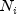denote the sum of the frequencies of the observations in group i, and let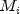denote the number of observations in group i. Then, if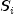denotes the within-group i covariance matrix,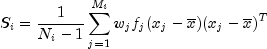where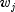is the weight of the j-th observation in group i,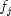is its frequency,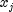is the j-th observation column vector (in group i), and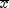denotes the mean vector of the observations in group i. The mean vectors are computed as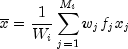where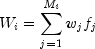Given the means and the covariance matrices, the linear discriminant function for group i is computed as: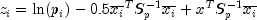where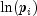is the natural log of the prior probability for the i-th group, x is the observation to be classified, and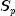denotes the pooled covariance matrix.

Let S denote either the pooled covariance matrix or one of the within-group covariance matrices. (S will be the pooled covariance matrix in linear discrimination, andotherwise.) The Mahalanobis distance between group i and group j is computed as: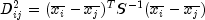Finally, the asymptotic chi-squared test for the equality of covariance matrices is computed as follows (Morrison 1976, page 252):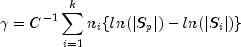where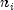is the number of degrees of freedom in the i-th sample covariance matrix,is the number of groups, and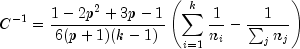whereis the number of variables.

The estimated posterior probability of each observation x belonging to group i is computed using the prior probabilities and the sample mean vectors and estimated covariance matrices under a multivariate normal assumption. Under quadratic discrimination, the within-group covariance matrices are used to compute the estimated posterior probabilities. The estimated posterior probability of an observation x belonging to group i is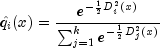where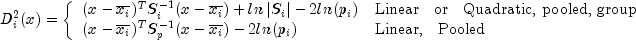For the leaving-out-one method of classification, the sample mean vector and sample covariance matrices in the formula for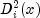are adjusted so as to remove the observation x from their computation. For linear discrimination, the linear discriminant function coefficients are actually used to compute the same posterior probabilities.

Using the posterior probabilities, each observation in x is classified into a group; the result is tabulated in the matrix returned by `getClassTable` and saved in the vector returned by `getClassMembership`. If a group variable is provided and the group number is out of range, the classification table is not altered at this stage. If the reclassification method is specified, then all observations with no missing values are classified. When the leaving-out-one method is used, observations with invalid group numbers, weights, frequencies or classification variables are not classified. Regardless of the frequency, a 1 is added (or subtracted) from the classification table for each row of `x` that is classified and contains a valid group number. When the leaving-out-one method is used, adjustment is made to the posterior probabilities to remove the effect of the observation in the classification rule. In this adjustment, each observation is presumed to have a weight ofand a frequency of 1.0. See Lachenbruch (1975, page 36) for the required adjustment.

Discriminant Analysis Example
• ### Nested Class Summary

Nested Classes
Modifier and Type Class and Description
`static class ` `DiscriminantAnalysis.CovarianceSingularException`
The variance-covariance matrix is singular.
`static class ` `DiscriminantAnalysis.EmptyGroupException`
There are no observations in a group.
`static class ` `DiscriminantAnalysis.SumOfWeightsNegException`
The sum of the weights have become negative.
• ### Field Summary

Fields
Modifier and Type Field and Description
`static int` `LEAVE_OUT_ONE`
Indicates leave-out-one classification method.
`static int` `LINEAR`
Indicates a linear discrimination method.
`static int` `POOLED`
Indicates pooled covariances computation.
`static int` `POOLED_GROUP`
Indicates pooled, group covariances computation.
`static int` `PRIOR_EQUAL`
Indicates prior equal probabilities.
`static int` `PRIOR_PROPORTIONAL`
Indicates prior proportional probabilities.
`static int` `QUADRATIC`
`static int` `RECLASSIFICATION`
Indicates reclassification classification method.
• ### Constructor Summary

Constructors
Constructor and Description
```DiscriminantAnalysis(int nVariables, int nGroups)```
Constructs a `DiscriminantAnalysis`.
• ### Method Summary

Methods
Modifier and Type Method and Description
`void` `classify(double[][] x)`
Classify a set of observations using the linear or quadratic discriminant functions generated during the training process.
`void` ```classify(double[][] x, int[] varIndex)```
Classify a set of observations using the linear or quadratic discriminant functions generated during the training process.
`void` ```classify(double[][] x, int[] frequencies, double[] weights)```
Classify a set of observations and associated frequencies and weights using the linear or quadratic discriminant functions generated during the training process.
`void` ```classify(double[][] x, int[] group, int[] varIndex)```
Classify a set of observations and compare against known groups using the linear or quadratic discriminant functions generated during the training process.
`void` ```classify(double[][] x, int[] varIndex, int[] frequencies, double[] weights)```
Classify a set of observations and associated frequencies and weights using the linear or quadratic discriminant functions generated during the training process.
`void` ```classify(double[][] x, int[] group, int[] varIndex, int[] frequencies, double[] weights)```
Classify a set of observations, associated frequencies and weights, and compare against known groups using the linear or quadratic discriminant functions generated during the training process.
`void` ```downdate(double[][] x, int[] group)```
Removes a set of observations from the discriminant functions.
`void` ```downdate(double[][] x, int[] group, int[] varIndex)```
Removes a set of observations from the discriminant functions.
`void` ```downdate(double[][] x, int[] group, int[] frequencies, double[] weights)```
Removes a set of observations and associated frequencies and weights from the discriminant functions.
`void` ```downdate(double[][] x, int[] group, int[] varIndex, int[] frequencies, double[] weights)```
Removes a set of observations and associated frequencies and weights from the discriminant functions.
`int[]` `getClassMembership()`
Returns the group number to which the observation was classified.
`double[][]` `getClassTable()`
Returns the classification table.
`double[][]` `getCoefficients()`
Returns the linear discriminant function coefficients.
`double[][][]` `getCovariance()`
Returns the array of covariances.
`int[]` `getGroupCounts()`
Returns the group counts.
`double[][]` `getMahalanobis()`
Returns the Mahalanobis distances between the group means.
`double[][]` `getMeans()`
Returns the variable means.
`int` `getNumberOfRowsMissing()`
Returns the number of rows of data encountered containing missing values (`Double.NaN`).
`double[]` `getPrior()`
Returns the prior probabilities.
`double[][]` `getProbability()`
Returns the posterior probabilities for each observation.
`double[]` `getStatistics()`
Returns statistics.
`void` `setClassificationMethod(int method)`
Specifies the classification method to be either reclassification or leave-out-one.
`void` `setCovarianceComputation(int type)`
Specifies the covariance matrix computation to be either pooled or pooled, group.
`void` `setDiscriminationMethod(int method)`
Specifies the discrimination method used to be either linear or quadratic discrimination.
`void` `setPrior(double[] prior)`
Specifies user supplied prior probabilities.
`void` `setPrior(int prior)`
Specifies the prior probabilities to be calculated as either equal or proportional priors.
`void` ```update(double[][] x, int[] group)```
Trains a set of observations and associated frequencies and weights by performing a linear or quadratic discriminant function analysis among several known groups.
`void` ```update(double[][] x, int[] group, int[] varIndex)```
Trains a set of observations and associated frequencies and weights by performing a linear or quadratic discriminant function analysis among several known groups.
`void` ```update(double[][] x, int[] group, int[] frequencies, double[] weights)```
Trains a set of observations and associated frequencies and weights by performing a linear or quadratic discriminant function analysis among several known groups.
`void` ```update(double[][] x, int[] group, int[] varIndex, int[] frequencies, double[] weights)```
Trains a set of observations and associated frequencies and weights by performing a linear or quadratic discriminant function analysis among several known groups.
• ### Methods inherited from class java.lang.Object

`clone, equals, finalize, getClass, hashCode, notify, notifyAll, toString, wait, wait, wait`
• ### Field Detail

• #### LEAVE_OUT_ONE

`public static final int LEAVE_OUT_ONE`
Indicates leave-out-one classification method.
Constant Field Values
• #### LINEAR

`public static final int LINEAR`
Indicates a linear discrimination method.
Constant Field Values
• #### POOLED

`public static final int POOLED`
Indicates pooled covariances computation.
Constant Field Values
• #### POOLED_GROUP

`public static final int POOLED_GROUP`
Indicates pooled, group covariances computation.
Constant Field Values
• #### PRIOR_EQUAL

`public static final int PRIOR_EQUAL`
Indicates prior equal probabilities.
Constant Field Values
• #### PRIOR_PROPORTIONAL

`public static final int PRIOR_PROPORTIONAL`
Indicates prior proportional probabilities.
Constant Field Values

`public static final int QUADRATIC`
Constant Field Values
• #### RECLASSIFICATION

`public static final int RECLASSIFICATION`
Indicates reclassification classification method.
Constant Field Values
• ### Constructor Detail

• #### DiscriminantAnalysis

```public DiscriminantAnalysis(int nVariables,
int nGroups)```
Constructs a `DiscriminantAnalysis`.
Parameters:
`nVariables` - an `int` representing the number of variables to be used in the discrimination
`nGroups` - an `int` representing the number of groups in the data
• ### Method Detail

• #### classify

```public void classify(double[][] x)
throws DiscriminantAnalysis.SumOfWeightsNegException,
DiscriminantAnalysis.EmptyGroupException,
DiscriminantAnalysis.CovarianceSingularException```
Classify a set of observations using the linear or quadratic discriminant functions generated during the training process.
Parameters:
`x` - a `double` matrix containing the observations with at least `nVariables` columns. The first `nVariables` columns correspond to the variables. Reclassification does not require group numbers be present. Any additional columns will be ignored.
Throws:
`IllegalStateException` - is thrown if the leave-out-one classification method is chosen.
`DiscriminantAnalysis.SumOfWeightsNegException` - is thrown when the sum of the weights have become negative.
`DiscriminantAnalysis.EmptyGroupException` - is thrown when there are no observations in a group.
`DiscriminantAnalysis.CovarianceSingularException` - is thrown when the variance-covariance matrix is singular.
• #### classify

```public void classify(double[][] x,
int[] varIndex)
throws DiscriminantAnalysis.SumOfWeightsNegException,
DiscriminantAnalysis.EmptyGroupException,
DiscriminantAnalysis.CovarianceSingularException```
Classify a set of observations using the linear or quadratic discriminant functions generated during the training process.
Parameters:
`x` - a `double` matrix containing the observations with at least `nVariables` columns. The columns indicated in `varIndex` correspond to the variables. Reclassification does not require group numbers be present. Additional columns will be ignored.
`varIndex` - an `int` array containing the column indices in `x` that correspond to the variables to be used in the analysis
Throws:
`IllegalStateException` - is thrown if the leave-out-one classification method is chosen.
`DiscriminantAnalysis.SumOfWeightsNegException` - is thrown when the sum of the weights have become negative.
`DiscriminantAnalysis.EmptyGroupException` - is thrown when there are no observations in a group.
`DiscriminantAnalysis.CovarianceSingularException` - is thrown when the variance-covariance matrix is singular.
• #### classify

```public void classify(double[][] x,
int[] frequencies,
double[] weights)
throws DiscriminantAnalysis.SumOfWeightsNegException,
DiscriminantAnalysis.EmptyGroupException,
DiscriminantAnalysis.CovarianceSingularException```
Classify a set of observations and associated frequencies and weights using the linear or quadratic discriminant functions generated during the training process.
Parameters:
`x` - a `double` matrix containing the observations with at least `nVariables` columns. The first `nVariables` columns correspond to the variables. Reclassification does not require group numbers be present. Any additional columns will be ignored.
`frequencies` - an `int` array containing the associated frequencies for each observation
`weights` - a `double` array containing the associated weights for each observation
Throws:
`IllegalStateException` - is thrown if the leave-out-one classification method is chosen
`DiscriminantAnalysis.SumOfWeightsNegException` - is thrown when the sum of the weights have become negative
`DiscriminantAnalysis.EmptyGroupException` - is thrown when there are no observations in a group
`DiscriminantAnalysis.CovarianceSingularException` - is thrown when the variance-covariance matrix is singular
• #### classify

```public void classify(double[][] x,
int[] group,
int[] varIndex)
throws DiscriminantAnalysis.SumOfWeightsNegException,
DiscriminantAnalysis.EmptyGroupException,
DiscriminantAnalysis.CovarianceSingularException```
Classify a set of observations and compare against known groups using the linear or quadratic discriminant functions generated during the training process.
Parameters:
`x` - a `double` matrix containing the observations with at least `nVariables` columns. The columns indicated in `varIndex` correspond to the variables. Any additional columns will be ignored.
`group` - an `int` array containing the group numbers. The groups must be numbered 1,2, ..., `nGroups` for each observation.
`varIndex` - an `int` array containing the column indices in `x` that correspond to the variables to be used in the analysis
Throws:
`DiscriminantAnalysis.SumOfWeightsNegException` - is thrown when the sum of the weights have become negative
`DiscriminantAnalysis.EmptyGroupException` - is thrown when there are no observations in a group
`DiscriminantAnalysis.CovarianceSingularException` - is thrown when the variance-covariance matrix is singular
• #### classify

```public void classify(double[][] x,
int[] varIndex,
int[] frequencies,
double[] weights)
throws DiscriminantAnalysis.SumOfWeightsNegException,
DiscriminantAnalysis.EmptyGroupException,
DiscriminantAnalysis.CovarianceSingularException```
Classify a set of observations and associated frequencies and weights using the linear or quadratic discriminant functions generated during the training process.
Parameters:
`x` - a `double` matrix containing the observations with at least `nVariables` columns. The columns indicated in `varIndex` correspond to the variables. Reclassification does not require group numbers be present. Additional columns in `x` will be ignored.
`varIndex` - an `int` array containing the column indices in `x` that correspond to the variables to be used in the analysis
`frequencies` - an `int` array containing the associated frequencies for each observation
`weights` - a `double` array containing the associated weights for each observation
Throws:
`IllegalStateException` - is thrown if the leave-out-one classification method is chosen
`DiscriminantAnalysis.SumOfWeightsNegException` - is thrown when the sum of the weights have become negative
`DiscriminantAnalysis.EmptyGroupException` - is thrown when there are no observations in a group
`DiscriminantAnalysis.CovarianceSingularException` - is thrown when the variance-covariance matrix is singular
• #### classify

```public void classify(double[][] x,
int[] group,
int[] varIndex,
int[] frequencies,
double[] weights)
throws DiscriminantAnalysis.SumOfWeightsNegException,
DiscriminantAnalysis.EmptyGroupException,
DiscriminantAnalysis.CovarianceSingularException```
Classify a set of observations, associated frequencies and weights, and compare against known groups using the linear or quadratic discriminant functions generated during the training process.
Parameters:
`x` - a `double` matrix containing the observations with at least `nVariables` columns. The columns indicated in `varIndex` correspond to the variables. Additional columns are ignored.
`group` - an `int` array containing the group numbers. The groups must be numbered 1,2, ..., `nGroups` for each observation.
`varIndex` - an `int` array containing the column indices in `x` that correspond to the variables to be used in the analysis
`frequencies` - an `int` array containing the associated frequencies for each observation
`weights` - a `double` array containing the associated weights for each observation
Throws:
`DiscriminantAnalysis.SumOfWeightsNegException` - is thrown when the sum of the weights have become negative
`DiscriminantAnalysis.EmptyGroupException`
`DiscriminantAnalysis.CovarianceSingularException`
• #### downdate

```public void downdate(double[][] x,
int[] group)
throws DiscriminantAnalysis.SumOfWeightsNegException```
Removes a set of observations from the discriminant functions.
Parameters:
`x` - a `double` matrix containing the observations to be removed, with at least `nVariables` columns. The first `nVariables` columns correspond to the variables. Any additional columns will be ignored.
`group` - an `int` array containing the group numbers. The groups must be numbered 1,2, ..., `nGroups` for each observation.
Throws:
`DiscriminantAnalysis.SumOfWeightsNegException` - is thrown when the sum of the weights have become negative.
• #### downdate

```public void downdate(double[][] x,
int[] group,
int[] varIndex)
throws DiscriminantAnalysis.SumOfWeightsNegException```
Removes a set of observations from the discriminant functions.
Parameters:
`x` - a `double` matrix containing the observations to be removed, with at least `nVariables` columns. The columns indicated in `varIndex` correspond to the variables.
`group` - an `int` array containing the group numbers. The groups must be numbered 1,2, ..., `nGroups` for each observation.
`varIndex` - an `int` array containing the column indices in `x` that correspond to the variables to be used in the analysis
Throws:
`DiscriminantAnalysis.SumOfWeightsNegException` - is thrown when the sum of the weights have become negative.
• #### downdate

```public void downdate(double[][] x,
int[] group,
int[] frequencies,
double[] weights)
throws DiscriminantAnalysis.SumOfWeightsNegException```
Removes a set of observations and associated frequencies and weights from the discriminant functions.
Parameters:
`x` - a `double` matrix containing the observations to be removed, with at least `nVariables` columns. The columns indicated in `varIndex` correspond to the variables.
`group` - an `int` array containing the group numbers. The groups must be numbered 1,2, ..., `nGroups` for each observation.
`frequencies` - an `int` array containing the associated frequencies for each observation
`weights` - a `double` array containing the associated weights for each observation
Throws:
`DiscriminantAnalysis.SumOfWeightsNegException` - is thrown when the sum of the weights have become negative.
• #### downdate

```public void downdate(double[][] x,
int[] group,
int[] varIndex,
int[] frequencies,
double[] weights)
throws DiscriminantAnalysis.SumOfWeightsNegException```
Removes a set of observations and associated frequencies and weights from the discriminant functions.
Parameters:
`x` - a `double` matrix containing the observations to be removed, with at least `nVariables` columns. The columns indicated in `varIndex` correspond to the variables.
`group` - an `int` array containing the group numbers. The groups must be numbered 1,2, ..., `nGroups` for each observation.
`varIndex` - an `int` array containing the column indices in `x` that correspond to the variables to be used in the analysis
`frequencies` - an `int` array containing the associated frequencies for each observation
`weights` - a `double` array containing the associated weights for each observation
Throws:
`DiscriminantAnalysis.SumOfWeightsNegException` - is thrown when the sum of the weights have become negative.
• #### getClassMembership

`public int[] getClassMembership()`
Returns the group number to which the observation was classified.
Returns:
an `int` array containing the group to which the observation was classified. If an observation has an invalid group number, frequency, or weight when the leaving-out-one method has been specified, then the observation is not classified and the corresponding elements of the array are set to zero. Note this will return the classmembership of the last set of observations classified.
Throws:
`IllegalStateException` - is thrown if no data has been classified.
• #### getClassTable

`public double[][] getClassTable()`
Returns the classification table.
Returns:
an `nGroups` by `nGroups` `double` matrix containing the classification table. The accumulation of each observation that is classified and has a group number equal to 1, 2, ..., `nGroups` is entered into the table. If a known group is provided, the rows of the table correspond to the known group membership. The columns refer to the group to which the observation was classified. If a known group is not provided, the table will only contain the accumulated classified groups in the column coresponding to the group to which the observation was classified.
Throws:
`IllegalStateException` - is thrown if no data has been classified.
• #### getCoefficients

```public double[][] getCoefficients()
throws DiscriminantAnalysis.EmptyGroupException,
DiscriminantAnalysis.CovarianceSingularException```
Returns the linear discriminant function coefficients.
Returns:
an `nGroups` by `nVariables` `double` matrix containing the linear discriminant function coefficients. The first column of the matrix contains the constant term, and the remaining columns contain the variable coefficients. The i-th row of the returned matrix corresponds to group i. The coefficients are always computed as linear discriminant function coefficients even when quadratic discrimination is specified.
Throws:
`DiscriminantAnalysis.EmptyGroupException` - is thrown when there are no observations in a group.
`DiscriminantAnalysis.CovarianceSingularException` - is thrown when the variance-covariance matrix is singular.
• #### getCovariance

```public double[][][] getCovariance()
throws DiscriminantAnalysis.EmptyGroupException,
DiscriminantAnalysis.CovarianceSingularException```
Returns the array of covariances.
Returns:
a g by `nVariables` by `nVariables`   `double` array containing the covariances. Where, g = `nGroups`+1 if pooled, group covariance computation is specified or g=1 if pooled covariance computation is specified. When pooled only covariance matrices are computed, the within-group covariance matrices are not computed. The pooled covariance matrix is always computed and is returned as the g-th covariance matrix.

If this method is invoked before classification, the unscaled covariance matrix will be returned.

Throws:
`DiscriminantAnalysis.EmptyGroupException` - is thrown when there are no observations in a group.
`DiscriminantAnalysis.CovarianceSingularException` - is thrown when the variance-covariance matrix is singular.
• #### getGroupCounts

`public int[] getGroupCounts()`
Returns the group counts.
Returns:
an `int` array of length `nGroups` containing the number of observations in each group. If an update has not preceeded the invocation of this method, an array of all zeros will be returned.
• #### getMahalanobis

```public double[][] getMahalanobis()
throws DiscriminantAnalysis.EmptyGroupException,
DiscriminantAnalysis.CovarianceSingularException```
Returns the Mahalanobis distances between the group means.
Returns:
an `nGroups` by `nGroups`   `double` matrix containing the Mahalanobis distances between the group means. For linear discrimination, the Mahalanobis distance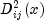between group means i and j is computed using the within covariance matrix for group i in place of the pooled covariance matrix.
Throws:
`DiscriminantAnalysis.EmptyGroupException` - is thrown when there are no observations in a group.
`DiscriminantAnalysis.CovarianceSingularException` - is thrown when the variance-covariance matrix is singular.
• #### getMeans

```public double[][] getMeans()
throws DiscriminantAnalysis.EmptyGroupException,
DiscriminantAnalysis.CovarianceSingularException```
Returns the variable means.
Returns:
an `nGroups` by `nVariables` `double` matrix containing the variable means. The i-th row contains the variable means for group i.

If this method is invoked before classification, the unscaled means will be returned.

Throws:
`DiscriminantAnalysis.EmptyGroupException` - is thrown when there are no observations in a group.
`DiscriminantAnalysis.CovarianceSingularException` - is thrown when the variance-covariance matrix is singular.
• #### getNumberOfRowsMissing

`public int getNumberOfRowsMissing()`
Returns the number of rows of data encountered containing missing values (`Double.NaN`).
Returns:
an `int` representing the number of rows of data encountered containing missing values (`Double.NaN`) for the classification, group, weight, and/or frequency variables. If a row of data contains a missing value (`Double.NaN`) for any of these variables, that row is excluded from the computations.
• #### getPrior

`public double[] getPrior()`
Returns the prior probabilities.
Returns:
a `double` array of length `nGroups` containing the prior probabilities for each group.
• #### getProbability

`public double[][] getProbability()`
Returns the posterior probabilities for each observation.
Returns:
an `x.length` by `nGroups`   `double` matrix containing the posterior probabilities for each observation. Note this will return the probabilities of the last set of observations classified.
Throws:
`IllegalStateException` - is thrown if no data has been classified.
• #### getStatistics

```public double[] getStatistics()
throws DiscriminantAnalysis.EmptyGroupException,
DiscriminantAnalysis.CovarianceSingularException```
Returns statistics.
Returns:
a `double` array containing output statistics.  index Description 0 Sum of the degrees of freedom for the within-covariance matrices. 1 Chi-squared statistic. 2 The degrees of freedom in the chi-squared statistic. 3 Probability of a greater chi-squared, respectively, of a test of the homogeneity of the within-covariance matrices. (Not computed when the pooled only covariance matrix is computed). 4 thru (4+`nGroups`) Log of the determinant of each group's covariance matrix (not computed when the pooled only covariance matrix is computed) and of the pooled covariance matrix. Last (`nGroups + 1`) elements Sum of the weights within each group. Last element Sum of the weights in all groups.
Throws:
`DiscriminantAnalysis.EmptyGroupException` - is thrown when there are no observations in a group.
`DiscriminantAnalysis.CovarianceSingularException` - is thrown when the variance-covariance matrix is singular.
• #### setClassificationMethod

`public void setClassificationMethod(int method)`
Specifies the classification method to be either reclassification or leave-out-one.
Parameters:
`method` - an `int` indicating the method of classification. Use class member `RECLASSIFICATION` or `LEAVE_OUT_ONE`. By default, the `RECLASSIFICATION` method is used.
• #### setCovarianceComputation

`public void setCovarianceComputation(int type)`
Specifies the covariance matrix computation to be either pooled or pooled, group.
Parameters:
`type` - an `int` scalar indicating the type of covariance matrices to be computed. Use class member `POOLED` or `POOLED_GROUP`. By default, `POOLED_GROUP` is used.
• #### setDiscriminationMethod

`public void setDiscriminationMethod(int method)`
Specifies the discrimination method used to be either linear or quadratic discrimination.
Parameters:
`method` - an `int` scalar indicating the method of discrimination. Use class member `LINEAR` or `QUADRATIC`. By default, the `LINEAR` method is used.
• #### setPrior

`public void setPrior(double[] prior)`
Specifies user supplied prior probabilities.
Parameters:
`prior` - a `double` vector of length `nGroups` containing the prior probabilities for each group. The elements of `prior` should sum to 1.0. If the values of `prior` are less than 1.0e-20, they will be converted to the `Math.log(1.0e-20)`. By default, the prior probablities are calculated to be equal, see `setPrior(int)`.
• #### setPrior

`public void setPrior(int prior)`
Specifies the prior probabilities to be calculated as either equal or proportional priors.
Parameters:
`prior` - an `int` specifying how to calculate prior probabilities as either equal or proportional prior probabilities. Use class member `PRIOR_EQUAL` to set equal prior probabilities, calculated as 1.0/`nGroups`. Use class member `PRIOR_PROPORTIONAL` to calculate the priors to be proportional to the sample size in each group. The sum of all prior probabilities is equal to 1.0. If the values calculated for the priors are less than 1.0e-20, they will be converted to the `Math.log(1.0e-20)`. Prior probabilities are used in calculating statistics, coefficients, Mahalanobis, and classification probabilities. By default, `PRIOR_EQUAL` is used.
• #### update

```public void update(double[][] x,
int[] group)
throws DiscriminantAnalysis.SumOfWeightsNegException```
Trains a set of observations and associated frequencies and weights by performing a linear or quadratic discriminant function analysis among several known groups.
Parameters:
`x` - a `double` matrix containing the observations with at least `nVariables` columns. The first `nVariables` correspond to the variables. Any additional columns will be ignored.
`group` - an `int` array containing the group numbers. The groups must be numbered
1,2, ..., `nGroups` for each observation.
Throws:
`DiscriminantAnalysis.SumOfWeightsNegException` - is thrown when the sum of the weights have become negative.
• #### update

```public void update(double[][] x,
int[] group,
int[] varIndex)
throws DiscriminantAnalysis.SumOfWeightsNegException```
Trains a set of observations and associated frequencies and weights by performing a linear or quadratic discriminant function analysis among several known groups.
Parameters:
`x` - a `double` matrix containing the observations with at least `nVariables` columns. The columns indicated in `varIndex` correspond to the variables. Any additional columns will be ignored.
`group` - an `int` array containing the group numbers. The groups must be numbered
1,2, ..., `nGroups` for each observation.
`varIndex` - an `int` array containing the column indices in `x` that correspond to the variables to be used in the analysis
Throws:
`DiscriminantAnalysis.SumOfWeightsNegException` - is thrown when the sum of the weights have become negative.
• #### update

```public void update(double[][] x,
int[] group,
int[] frequencies,
double[] weights)
throws DiscriminantAnalysis.SumOfWeightsNegException```
Trains a set of observations and associated frequencies and weights by performing a linear or quadratic discriminant function analysis among several known groups.
Parameters:
`x` - a `double` matrix containing the observations with at least `nVariables` columns. The first `nVariables` correspond to the variables. Any additional columns will be ignored.
`group` - an `int` array containing the group numbers. The groups must be numbered
1,2, ..., `nGroups` for each observation.
`frequencies` - an `int` array containing the associated frequencies for each observation
`weights` - a `double` array containing the associated weights for each observation
Throws:
`DiscriminantAnalysis.SumOfWeightsNegException` - is thrown when the sum of the weights have become negative.
• #### update

```public void update(double[][] x,
int[] group,
int[] varIndex,
int[] frequencies,
double[] weights)
throws DiscriminantAnalysis.SumOfWeightsNegException```
Trains a set of observations and associated frequencies and weights by performing a linear or quadratic discriminant function analysis among several known groups.
Parameters:
`x` - a `double` matrix containing the observations with at least `nVariables` columns. The columns indicated in `varIndex` correspond to the variables.
`group` - an `int` array containing the group numbers. The groups must be numbered
1,2, ..., `nGroups` for each observation.
`varIndex` - an `int` array containing the column indices in `x` that correspond to the variables to be used in the analysis
`frequencies` - an `int` array containing the associated frequencies for each observation
`weights` - a `double` array containing the associated weights for each observation
Throws:
`DiscriminantAnalysis.SumOfWeightsNegException` - is thrown when the sum of the weights have become negative.
JMSLTM Numerical Library 7.2.0